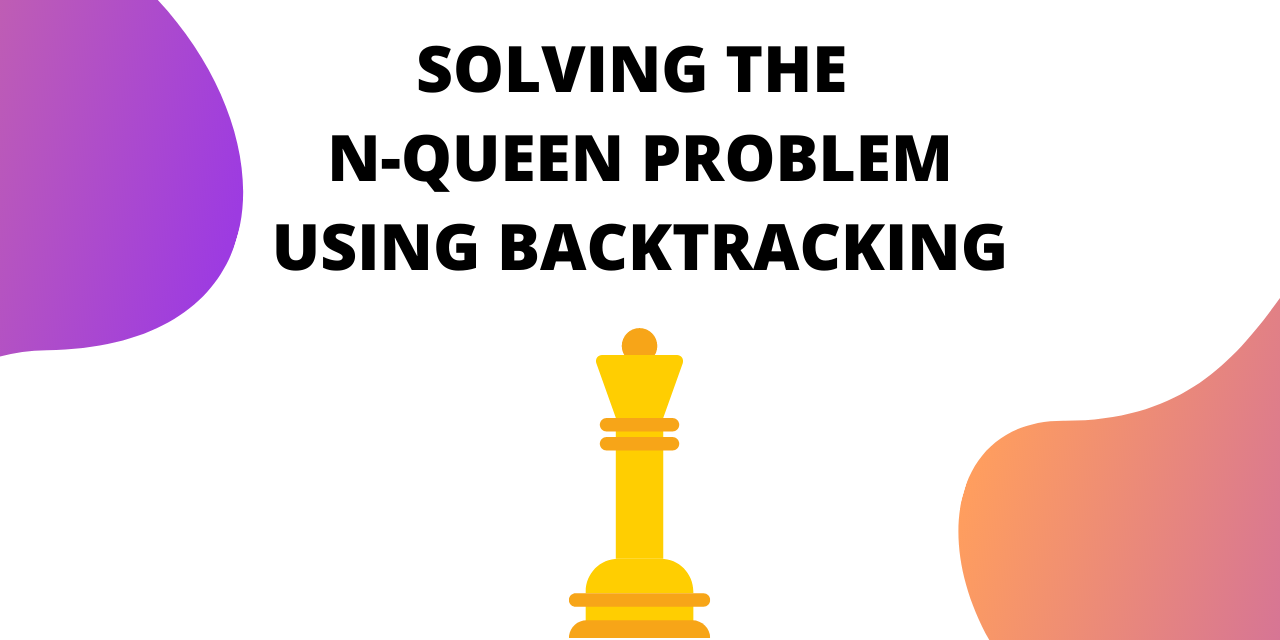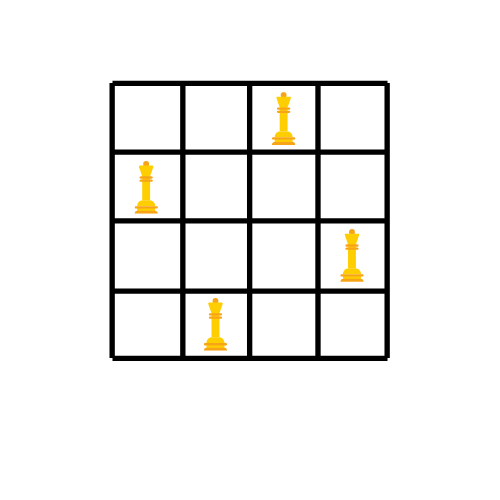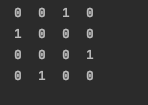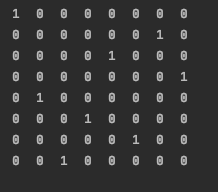# N-Queens problem using backtracking in Java/C++

Published on August 3, 2022By Jayant VermaWhile we believe that this content benefits our community, we have not yet thoroughly reviewed it. If you have any suggestions for improvements, please let us know by clicking the “report an issue“ button at the bottom of the tutorial.

If you love playing chess, you’ll enjoy learning about the N-queens problem. It is a good problem to understand backtracking.

## What is Backtracking?

In backtracking, we start with one pos­si­ble move out of many avail­able moves. We then try to solve the prob­lem.

If we are able to solve the prob­lem with the selected move then we will print the solu­tion. Else we will back­track and select some other move and try to solve it.

If none of the moves works out we claim that there is no solu­tion for the problem.

## What is the N-Queens Problem?

``````How can N queens be placed on an NxN chessboard so that no two of them attack each other?
``````

This problem is commonly seen for N=4 and N=8.

Let’s look at an example where N=4

Before solving the problem, you need to know about the movement of the queen in chess.

A queen can move any number of steps in any direction. The only constraint is that it can’t change its direction while it’s moving.

One thing that is clear by looking at the queen’s movement is that no two queens can be in the same row or column.

That allows us to place only one queen in each row and each column.

When N=4, the solution looks like :

But how do we get this arrangement?

## Solution to the N-Queens Problem

The way we try to solve this is by placing a queen at a position and trying to rule out the possibility of it being under attack. We place one queen in each row/column.

If we see that the queen is under attack at its chosen position, we try the next position.

If a queen is under attack at all the positions in a row, we backtrack and change the position of the queen placed prior to the current position.

We repeat this process of placing a queen and backtracking until all the N queens are placed successfully.

The step by step backtracking is shown as follows:

The red cross marks the positions which are under attack from a queen. Whenever we reach a state where we have a queen to place but all the positions in the rows are under attack, we backtrack.

This is not the only possible solution to the problem. If you move each queen one step forward in a clockwise manner, you get another solution.In this example we placed the queens according to rows, we can do the same thing column-wise also. In that case, each queen will belong to a column.

### Implementation of the N-Queens Problem in C++ and Java

Implementing N-Queens problem in C++:

``````#define N 4
#include <stdbool.h>
#include <stdio.h>
//function to print the solution
void printSolution(int board[N][N])
{
for (int i = 0; i < N; i++) {
for (int j = 0; j < N; j++)
printf(" %d ", board[i][j]);
printf("\n");
}
}

// function to check whether the position is safe or not
bool isSafe(int board[N][N], int row, int col)
{
int i, j;
for (i = 0; i < col; i++)
if (board[row][i])
return false;

for (i = row, j = col; i >= 0 && j >= 0; i--, j--)
if (board[i][j])
return false;
for (i = row, j = col; j >= 0 && i < N; i++, j--)
if (board[i][j])
return false;

return true;
}

// The function that solves the problem using backtracking
bool solveNQUtil(int board[N][N], int col)
{
if (col >= N)
return true;

for (int i = 0; i < N; i++) {
//if it is safe to place the queen at position i,col -> place it
if (isSafe(board, i, col)) {

board[i][col] = 1;

if (solveNQUtil(board, col + 1))
return true;

//backtrack if the above condition is false
board[i][col] = 0; // BACKTRACK
}
}

return false;
}

// driver program to test above function
int main()
{
int board[N][N] = { { 0, 0, 0, 0 },
{ 0, 0, 0, 0 },
{ 0, 0, 0, 0 },
{ 0, 0, 0, 0 } };

if (solveNQUtil(board, 0) == false) {
printf("Solution does not exist");
return 0;
}

printSolution(board);
return true;
return 0;
}
``````

Implementation of N-queens problem in Java:

``````package com.JournalDev;
public class Main {
static final int N = 4;

// print the final solution matrix
static void printSolution(int board[][])
{
for (int i = 0; i < N; i++) {
for (int j = 0; j < N; j++)
System.out.print(" " + board[i][j]
+ " ");
System.out.println();
}
}

// function to check whether the position is safe or not
static boolean isSafe(int board[][], int row, int col)
{
int i, j;
for (i = 0; i < col; i++)
if (board[row][i] == 1)
return false;

for (i = row, j = col; i >= 0 && j >= 0; i--, j--)
if (board[i][j] == 1)
return false;

for (i = row, j = col; j >= 0 && i < N; i++, j--)
if (board[i][j] == 1)
return false;

return true;
}

// The function that solves the problem using backtracking
public static boolean solveNQueen(int board[][], int col)
{
if (col >= N)
return true;

for (int i = 0; i < N; i++) {
//if it is safe to place the queen at position i,col -> place it
if (isSafe(board, i, col)) {
board[i][col] = 1;

if (solveNQueen(board, col + 1))
return true;

//backtrack if the above condition is false
board[i][col] = 0;
}
}
return false;
}

public static void main(String args[])
{
int board[][] = { { 0, 0, 0, 0 },
{ 0, 0, 0, 0 },
{ 0, 0, 0, 0 },
{ 0, 0, 0, 0 } };

if (!solveNQueen(board, 0)) {
System.out.print("Solution does not exist");
return;
}

printSolution(board);

}
}

``````
``````Output :
0  0  1  0
1  0  0  0
0  0  0  1
0  1  0  0
``````For N=8 the output is :

`````` 1  0  0  0  0  0  0  0
0  0  0  0  0  0  1  0
0  0  0  0  1  0  0  0
0  0  0  0  0  0  0  1
0  1  0  0  0  0  0  0
0  0  0  1  0  0  0  0
0  0  0  0  0  1  0  0
0  0  1  0  0  0  0  0
``````### Understanding the Code Implementation

To check whether a position is under attack or not we have created a function called isSafe.

The function returns true if the positions safe from any attack.

`````` static boolean isSafe(int board[][], int row, int col)
{
int i, j;
for (i = 0; i < col; i++)
if (board[row][i] == 1)
return false;

for (i = row, j = col; i >= 0 && j >= 0; i--, j--)
if (board[i][j] == 1)
return false;

for (i = row, j = col; j >= 0 && i < N; i++, j--)
if (board[i][j] == 1)
return false;

return true;
}
``````

The first for loop checks along the column, the second and third for loops check along the two diagonals.

The following piece of code is responsible for placing the queens at their position and backtracking. To mark the position of a queen we set that cell as 1 in the matrix. Before placing the queen, we call isSafe to make sure that the position is safe.

``````public static boolean solveNQueen(int board[][], int col)
{
if (col >= N)
return true;

for (int i = 0; i < N; i++) {
//if it is safe to place the queen at position i,col -> place it
if (isSafe(board, i, col)) {
board[i][col] = 1;

if (solveNQueen(board, col + 1))
return true;

//backtrack if the above condition is false
board[i][col] = 0;
}
}
return false;
}
``````

The recursive call passes the board and sets column to col+1. If the recursive call returns false, we backtrack by resetting the entry to 0.

## Conclusion

This is how you solve the N-Queen problem using backtracking. To learn more about backtracking try solving the sudoku problem.

Thanks for learning with the DigitalOcean Community. Check out our offerings for compute, storage, networking, and managed databases.Jayant Verma

author

#### Still looking for an answer?

Ask a questionSearch for more help

Click below to sign up and get \$200 of credit to try our products over 60 days!

### Popular Topics##### Hollie's Hub for Good

Working on improving health and education, reducing inequality, and spurring economic growth? We’d like to help.##### Become a contributor

You get paid; we donate to tech nonprofits.

##### Welcome to the developer cloud

DigitalOcean makes it simple to launch in the cloud and scale up as you grow – whether you’re running one virtual machine or ten thousand.© 2023 DigitalOcean, LLC.